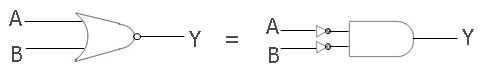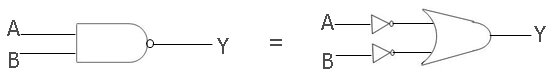# De Morgan's Theorem of Boolean Algebra

There are two theorems given by De Morgan's for boolean algebra that we are going to discuss in this article or tutorial.

## De Morgan's First Theorem

De Morgan's first theorem states that the complement of the sum equals the product of the complement.

### Boolean Expression of De Morgan's First Theorem

Here is the boolean expression of De Morgan's first theorem:

`(A+B)' = (A'.B')`

Here, A and B are the two binary variables. Binary variables are those in which both variables can hold either 0 or 1.

### Graphical Representation of De Morgan's First Theorem

Below is the symbol or graphical representation of De Morgan's first theorem:As you can see from the above figure, the first gate is a NOR gate, which is taking two inputs, A and B, and giving the output Y that will be equal to (A+B)'. And the second gate is the AND gate, but here there are two inputs A and B that are passing through a NOT gate and then enter the AND gate as input. Therefore, at the AND gate's input terminal, both the inputs will become A' and B', and therefore the output Y holds the value (A'.B').

### Proof of De Morgan's First Theorem using the Truth Table

Here is the truth table that identifies the proof of De Morgan's first theorem equation, which is (A+B)'=(A'.B'):

A B A' B' A+B (A+B)' A'.B'
0 0 1 1 0 1 1
0 1 1 0 1 0 0
1 0 0 1 1 0 0
1 1 0 0 1 0 0

As you can clearly see, all the corresponding row's values of (A+B)' and (A'.B') are same. Therefore, the first theorem of De Morgan is proved using the above truth table.

## De Morgan's Second Theorem

According to De Morgan's second theorem, the complement of produce equals the sum of the complement.

### Boolean Expression of De Morgan's Second Theorem

The boolean expression of De Morgan's second theorem is given below:

`(A.B)' = (A'+B')`

### Graphical Representation of De Morgan's Second Theorem

Here is the symbol or graphical representation of the second theorem given by De-Morgan:Here, the first gate is a NAND gate; there are two inputs, A and B, provided here, which give Y at the output and will be equal to (A.B)'. And the second gate is an OR gate, and the inputs A and B provided here are passed through a NOT gate; therefore, these two inputs become A' and B' at output, which will be the input for the OR gate that gives Y at output that will be equal to (A'+B').

### Proof of De Morgan's Second Theorem using Truth Table

Here is the truth table to demonstrate De Morgan's second theorem:

A B A' B' A.B (A.B)' A'+B'
0 0 1 1 0 1 1
0 1 1 0 0 1 1
1 0 0 1 0 1 1
1 1 0 0 1 0 0

Again, as you can see, (A.B)' holds the same value as (A'+B') holds in all corresponding rows. Therefore, De Morgan's second theorem is demonstrated using the above truth table.

Computer Fundamentals Quiz

« Previous Tutorial Next Tutorial »